# Ideal generated by two prime elements in a unique factorization domain may be proper and not prime

## Statement

It is possible to have a unique factorization domain$R$ and primes$p,q \in R$ such that$(p,q)$ is a proper ideal of$R$ that is not prime.

## Related facts

• Unique factorization is not prime-quotient-closed: If$R$ is a unique factorization domain, and$P$ is a prime ideal, the quotient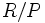$R/P$ need not be a unique factorization domain. Examples of this where the prime ideal$P$ is principal also give examples of ideals generated by two elements that are proper and not prime.

## Proof

### Example of a bivariate polynomial ring

Let$k$ be any field of characteristic not equal to$2$. Let <mah>R = k[x,y][/itex]. The polynomials$x$ and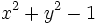$x^2 + y^2 - 1$ are irreducible in$R$, and hence prime. However, the ideal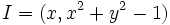$I = (x,x^2 + y^2 - 1)$ is not prime in$R$. To see this, note that$R/I =R/(x,y^2 - 1) = k[x,y]/(x,y^2 - 1) \cong k[y]/(y^2 - 1)$, which is not an integral domain, because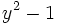$y^2 - 1$ is not irreducible.

### Example of a polynomial ring over the integers

Let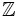$\mathbb{Z}$ be the ring of rational integers and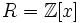$R = \mathbb{Z}[x]$. The elements$2, x^2 + 5$ are irreducible in$R$. However, the ideal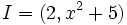$I = (2,x^2 + 5)$ is not a prime ideal in$R$. To see this, note that$I$ being prime is equivalent to the polynomial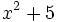$x^2 + 5$ being irreducible in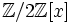$\mathbb{Z}/2\mathbb{Z}[x]$, which is not true since the polynomial reduces as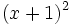$(x+1)^2$ modulo$2$.